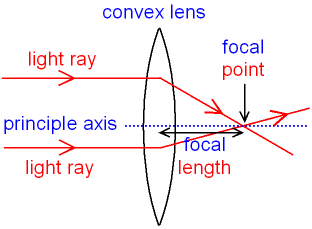gcsescience.com                                       26                                       gcsescience.com

Waves

What are Ray Diagrams?

Ray diagrams for a convex lens and a concave lens are different
but to draw any ray diagram you only need to know two things.

1. A ray passing through the centre of a lens
will go straight through.
2. A ray parallel to the principle axis of a lens
will go through the focal point.

What is the Principle Axis and the Focal Point of a Convex Lens?

The principle axis is a horizontal line going through the
centre of a lens (shown as the normal on the previous page).Any light ray parallel to the principle axis will be refracted,
change direction and cross the principle axis at the focal point.

What is the Focal Length of a Convex Lens?

The distance from the focal point to the centre of the lens
is called the focal length.

Almost parallel rays of light come from any object that is
a long way (more than 5 metres) from the lens (continued).

gcsescience.com       Physics Quiz       Index       Lens Quiz       gcsescience.com